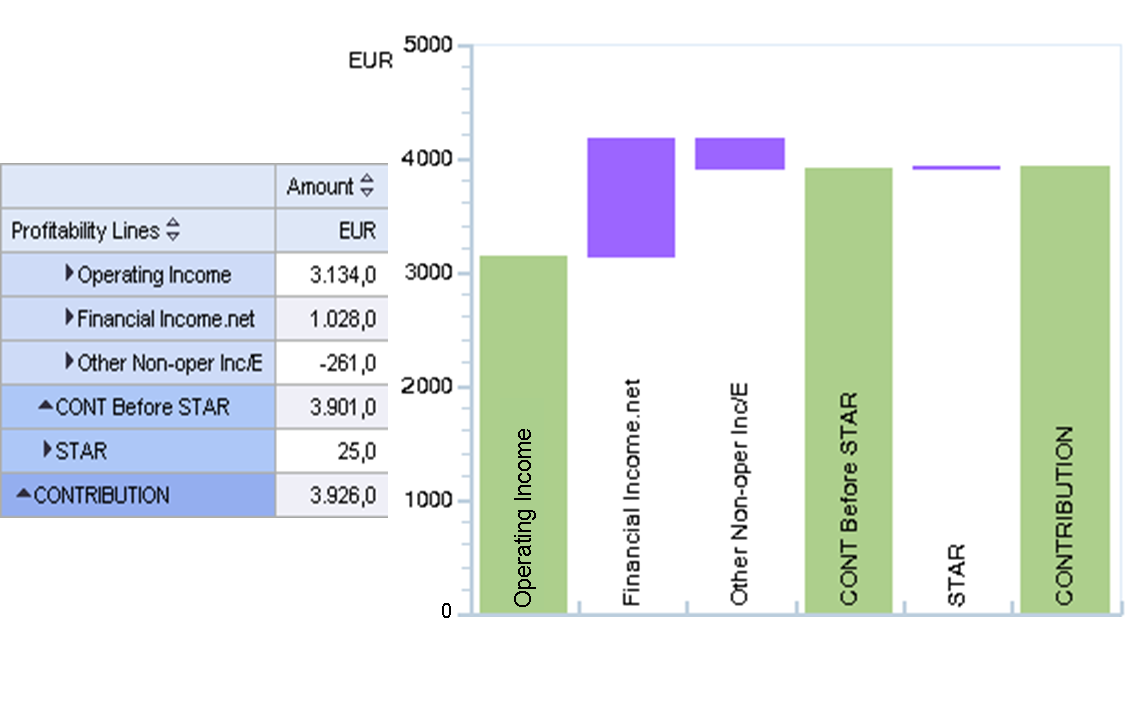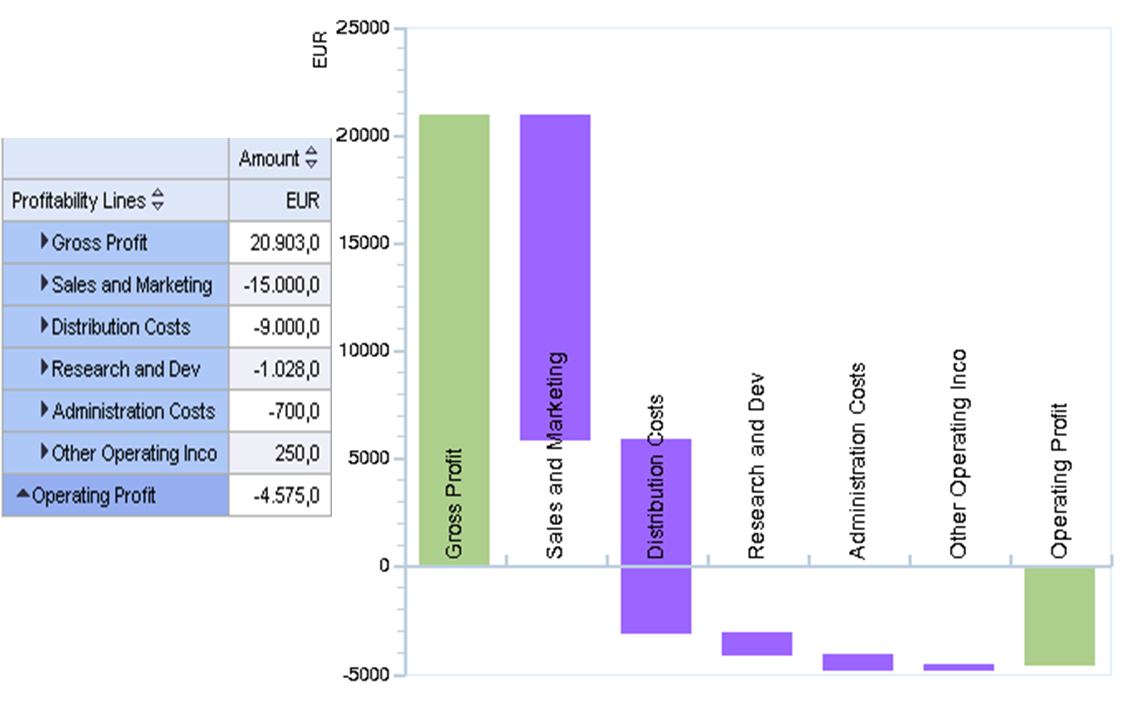Show TOC

# Class 5 Chart TypesThe chart type delta chart and its variant waterfall chart belong to class 5.

Delta Chart

A delta chart outlines the development of a total by displaying various interim values. Important to note is that these interim values are not displayed as subtotals, but as deltas.

The delta chart only deals with flat data tables; this means the interim values are individual items. If you have a hierarchical data table, that is, if the interim values are made up of multiple single items, the system automatically selects the waterfall chart variant to display the data.

Differences Between the Chart Types Delta Chart and Waterfall Chart

Delta Chart Waterfall Chart

Hierarchy in data table

No

Yes

Number of totals

2 (start value, end value)

2+n (start value, end value, and interim values, if applicable)

Example Table for Delta Chart

Profitability Line Earned Amount

Cost of Goods Sold

-41,500.00

Customer Rebates

-5,700.00

-5,000.00

Quantity Discount

-9,050.00

Returns

-4,325.00

Revenue

87,000.00

Overall Result

21,425.00

Tip

The example contains a table for a delta chart. The table does not contain a hierarchy (hierarchy nodes); a further drilldown is therefore not possible. The totals used in the chart are the items Cost of Goods Sold (start value), which is displayed on the debit side, and Revenue (end value), which is displayed on the credit side. All remaining values are interim values, which are displayed in the chart as deltas. The start and end values (including all interim values) are used to calculate the total sum (Overall Result).

Example for Delta ChartTip

The example includes the items Cost of Goods Soldand Revenue as totals (displayed in blue). The four interim values are displayed as deltas: Customer Rebates is therefore the difference between the previous value Cost of Goods Sold and the following value Overhead Costs, and so on.

Waterfall Chart as a Variant

The following applies to this variant:

• The start and end values are always totals.
• The data table is structured hierarchically. Individual interim values do not have any additional subsets - they are therefore not displayed as single items, but as hierarchy nodes. (See Example of a Waterfall Chart with Positive Valuesbelow.) These nodes can be expanded.
• All interim values without subsets are treated as deltas.
• All interim values with subsets (that is, nodes) that cannot be expanded, are treated as deltas.
• The last node in a hierarchy level is the total.

Example of a Waterfall Chart with Positive ValuesTip

The example shows the data table and the corresponding waterfall chart. Note that the start value CONTRIBUTION is displayed to the right of the chart in line with the structure of the table. CONTRIBUTION, CONT Before STAR, and Operating Income are displayed as totals.

Example of a Waterfall Chart with Positive and Negative Values